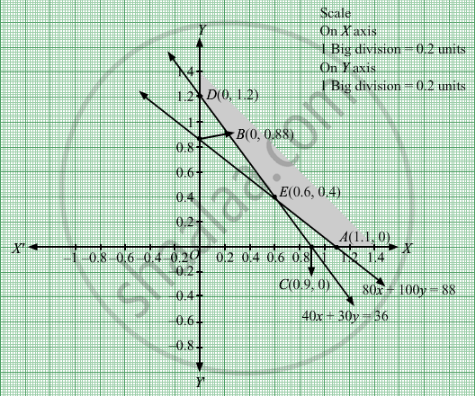# Kellogg is a New Cereal Formed of a Mixture of Bran and Rice that Contains at Least 88 Grams of Protein and at Least 36 Milligrams of Iron. - Mathematics

Sum

Kellogg is a new cereal formed of a mixture of bran and rice that contains at least 88 grams of protein and at least 36 milligrams of iron. Knowing that bran contains 80 grams of protein and 40 milligrams of iron per kilogram, and that rice contains 100 grams of protein and 30 milligrams of iron per kilogram, find the minimum cost of producing this new cereal if bran costs Rs 5 per kg and rice costs Rs 4 per kg

#### Solution

Let the cereal contain kg of bran and y kg of rice.
Therefore,

$x, y \geq 0$
The given information can be tabulated as follows
 Protein(grams) Iron(milligrams) Bran 80 40 Rice 100 30 minimum requirement 88 36

Bran and rice contains at least 88 grams of protein and at least 36 milligrams of iron.
Thus,the constraints are
$80x + 100y \geq 88$
$40x + 30y \geq 36$
It is given that bran costs Rs 5 per kg and rice costs Rs 4 per kg. Therefore, cost of kg of bran and y kg of rice is Rs 5x and Rs 4y respectively.
Hence, total profit is Rs (5x + 4y)
Let Z denote the total cost.
∴ z =  $5x + 4y$
Thus, the mathematical formulation of the given problem is Minimize $Z = 5x + 4y$
subject to
$80x + 100y \geq 88$
$40x + 30y \geq 36$
$x, y \geq 0$

First we will convert inequations into equations as follows :
80x + 100y = 60, 40x + 30y = 36, x = 0 and y = 0

Region represented by 80x + 100y ≥ 88:
The line 80x + 100y = 60 meets the coordinate axes at A(1.1, 0) and B(0, 0.88)respectively. By joining these points we obtain the line 80x + 100y = 60. Clearly (0,0) does not satisfies the 80x + 100y ≥ 88. So,the region which does not contains the origin represents the solution set of the inequation
80x + 100y ≥ 88.

Region represented by 40x + 30y ≥ 36:
The line 40x + 30y = 36 meets the coordinate axes at C(0.9, 0) and D(0, 1.2) respectively. By joining these points we obtain the line 40x + 30y = 36.
Clearly (0,0) does not satisfies the inequation 40x + 30y ≥ 36. So,the region which does not contains the origin represents the solution set of the inequation 40x + 30y ≥ 36.
Region represented by x ≥ 0 and y ≥ 0:
Since, every point in the first quadrant satisfies these inequations. So, the first quadrant is the region represented by the inequations x ≥ 0, and ≥ 0.
The feasible region determined by the system of constraints 80x + 100y ≥ 88, 40x + 30y≥ 36, x ≥ 0, and y ≥ 0 are as follows.
The feasible region determined by the system of constraints isThe corner points are D(0, 1.2), E(0.6, 0.4) and A(1.1, 0).
The values of Z at these corner points are as follows:
 Corner point Z = 5x + 4y D(0, 1.2) 4.8 E(0.6, 0.4) 4.6 A(1.1, 0) 5.5

The minimum value of Z is 4.6 which is attained at E(0.6, 0.4).
Hence, the minimum cost is Rs 4.6.

Concept: Graphical Method of Solving Linear Programming Problems
Is there an error in this question or solution?

#### APPEARS IN

RD Sharma Class 12 Maths
Chapter 30 Linear programming
Exercise 30.3 | Q 7 | Page 39